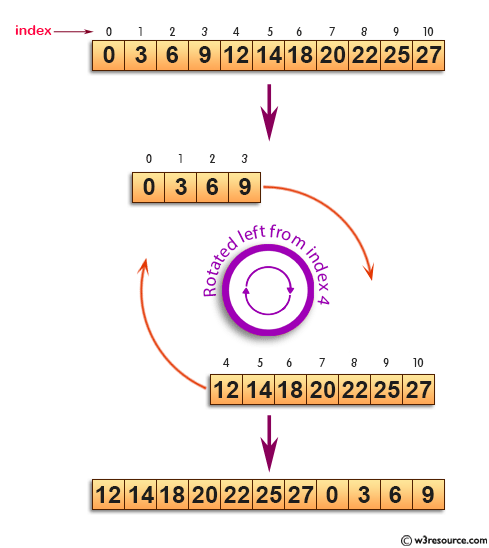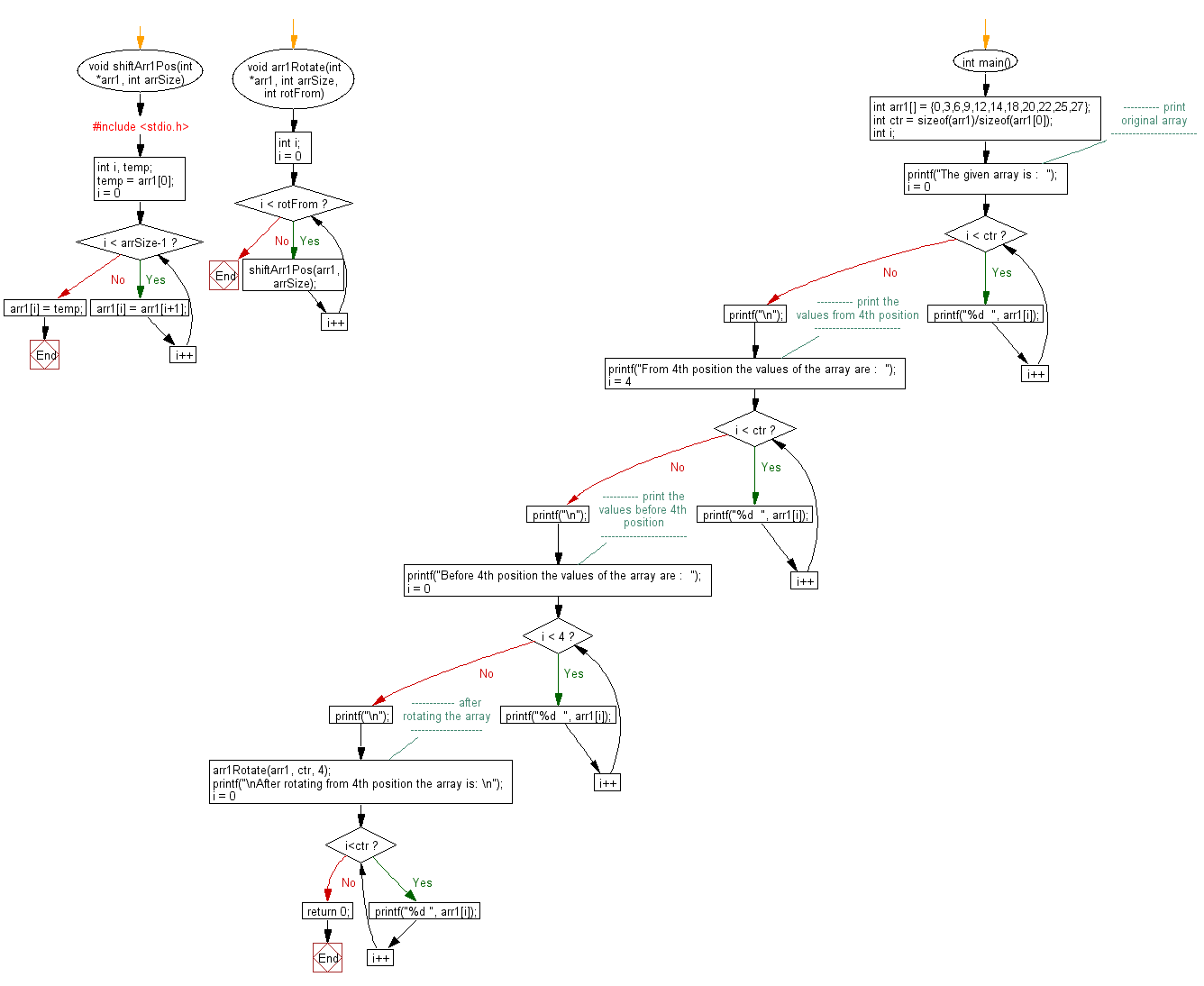﻿ C exercises: Rotate an array by N positions - w3resource# C Exercises: Rotate an array by N positions

## C Array: Exercise-39 with Solution

Write a program in C to rotate an array by N positions.
N.B.: The size of first array is (m+n) but only first m locations are populated remaining are empty. The second array is of size equal to n.

Pictorial Presentation:Sample Solution:

C Code:

``````#include <stdio.h>
void shiftArr1Pos(int *arr1, int arrSize)
{
int i, temp;
temp = arr1;
for(i = 0; i < arrSize-1; i++)
{
arr1[i] = arr1[i+1];
}
arr1[i] = temp;
}
void arr1Rotate(int *arr1, int arrSize, int rotFrom)
{
int i;
for(i = 0; i < rotFrom; i++)
{
shiftArr1Pos(arr1, arrSize);
}
return;
}
int main()
{
int arr1[] = {0,3,6,9,12,14,18,20,22,25,27};
int ctr = sizeof(arr1)/sizeof(arr1);
int i;
//---------- print original array ------------------------
printf("The given array is :  ");
for(i = 0; i < ctr; i++)
{
printf("%d  ", arr1[i]);
}
printf("\n");
//---------- print the values from 4th position ------------------------
printf("From 4th position the values of the array are :  ");
for(i = 4; i < ctr; i++)
{
printf("%d  ", arr1[i]);
}
printf("\n");
//---------- print the values before 4th position ------------------------
printf("Before 4th position the values of the array are :  ");
for(i = 0; i < 4; i++)
{
printf("%d  ", arr1[i]);
}
printf("\n");
//------------ after rotating the array --------------------
arr1Rotate(arr1, ctr, 4);
printf("\nAfter rotating from 4th position the array is: \n");
for(i = 0; i<ctr; i++)
{
printf("%d ", arr1[i]);
}
return 0;
}
```
```

Sample Output:

```The given array is :  0  3  6  9  12  14  18  20  22  25  27
From 4th position the values of the array are :  12  14  18  20  22  25  27
Before 4th position the values of the array are :  0  3  6  9

After rotating from 4th position the array is:
12 14 18 20 22 25 27 0 3 6 9
```

Flowchart:C Programming Code Editor:

Improve this sample solution and post your code through Disqus.

What is the difficulty level of this exercise?

﻿